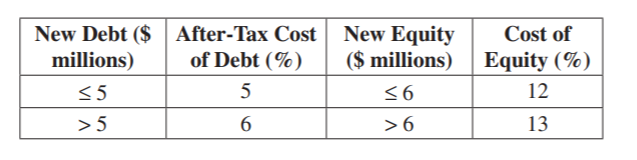Scan QR code or get instant email to install app

Question:

# An analyst gathers the following information about the cost of raising new debt and equity for a company:The company’s target capital structure is 70% equity and 30% debt. If the company raises $12m in financing, its cost of capital will be closest to:A 10.60%. explanation Break point for debt = 5 / 0.3 =$16.67m
Because the company will only be raising $12m (less than the break point for debt), its after‐tax cost of debt equals 5%. Break point for equity = 6 / 0.7 =$8.57m
Because the company will be raising \$12m (more than the break point for equity) its cost of equity equals 13%.
WACC = [0.13 x 0.7] + [0.05 x 0.3] = 10.6%.